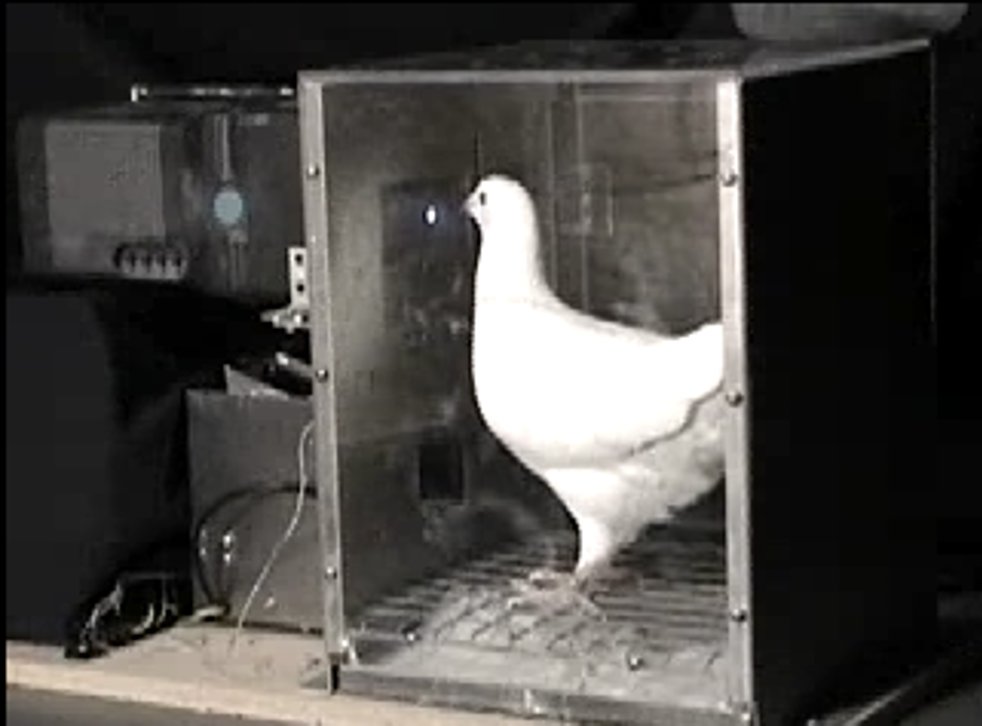# Reinforcement learning with more than one stimulus

Last time we developed a reinforcement learning model that could learn about the average payoffs from a single stimulus - either a slot machine or a fractal image. Even with this simple model we could already capture some interesting neural properties where the prediction error appears to describe (at least some of) the firing of dopamine neurons.
However, we've still got a ways to go before we have something we can apply to real-world learning problems. One particularly important limitation of the model so far is that we've only thought how to apply it to learning about one stimulus whose relevance for the reward is assumed to be known. For example, in the fractal example, we assumed that it was the predictive power of the fractal the monkeys were learning about. In the real world, there can be any number of stimuli that might be relevant for predicting a reward and whose relevance may not be clear. Thus a key next step in the model is to capture how humans and animals learn about more than one stimulus.
As a first step in this direction, we will consider the simplest possible case of more-than-one stimulus and try to model learning about two stimuli. This situation is already interesting because there are many ways in which two stimuli can be combined (e.g. one before the other, both at the same time, one there part of the time, etc ...).

## Three experiments with two stimuli

To help ground our discussion we will first consider three beahvioral experiments in which animals are trained to predict reward based on two stimuli. These experiments (or rather the effects they expose) are called: Overshadowing, Blocking, and Inhibition.